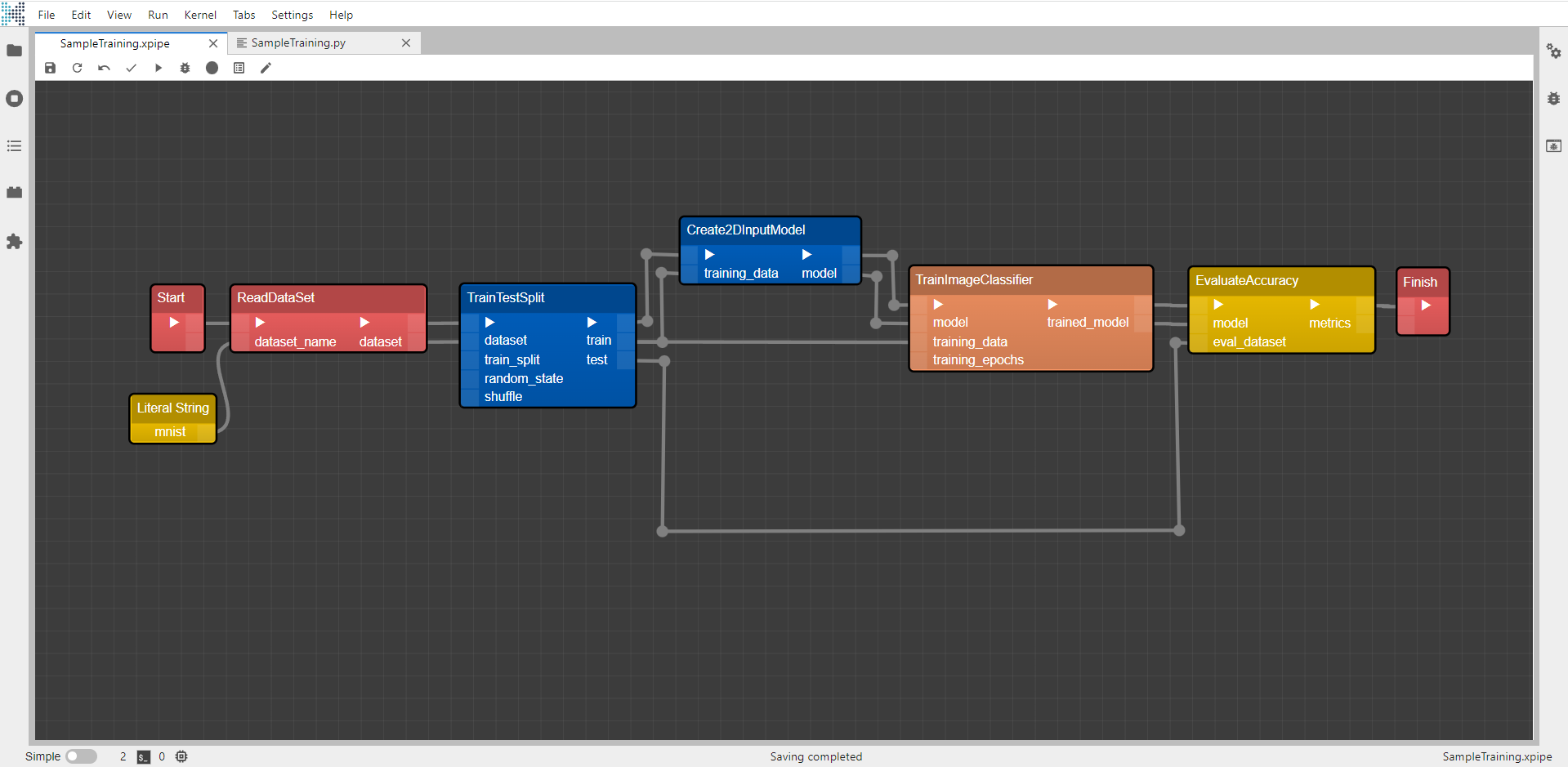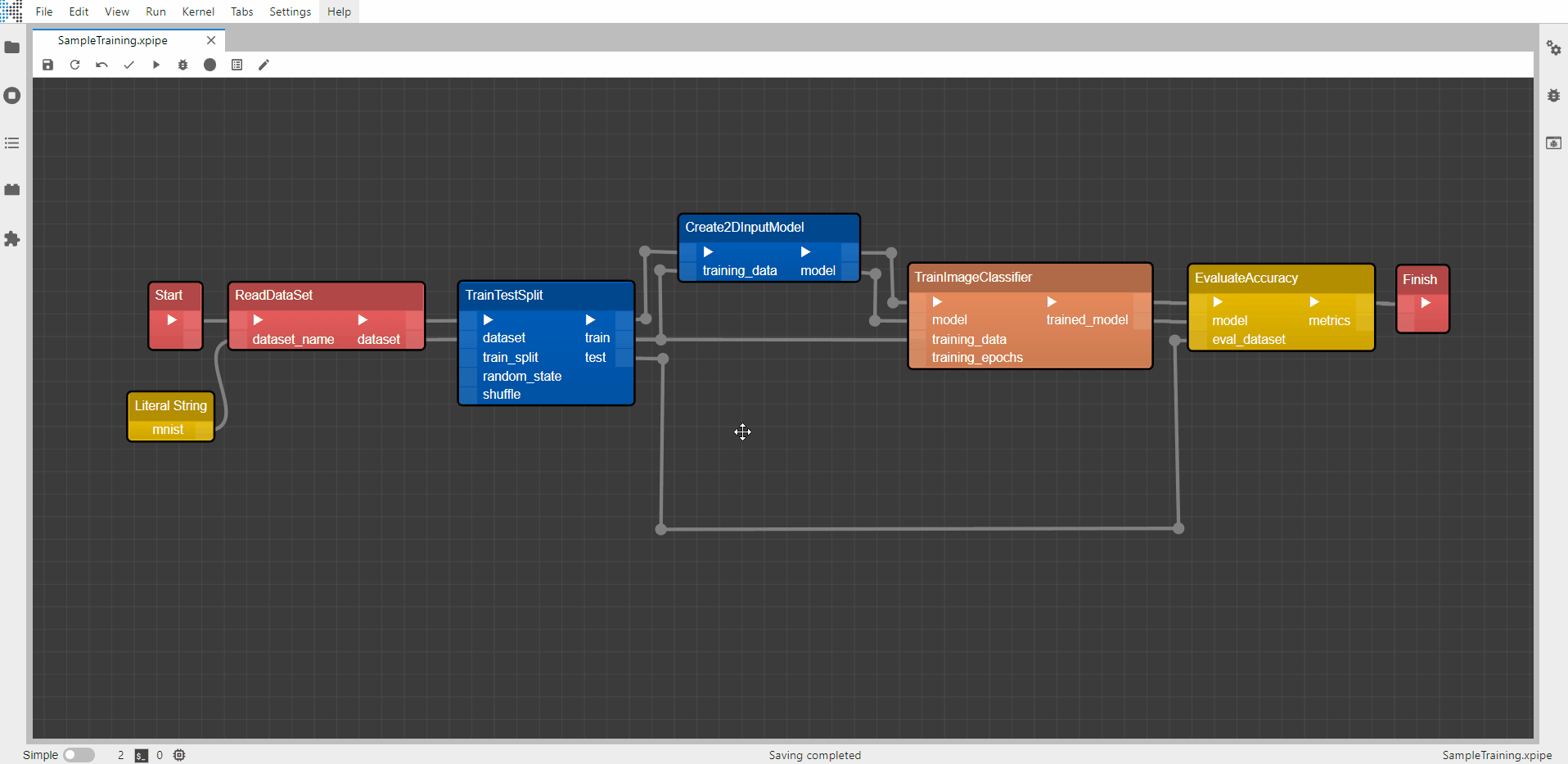# Literal & Hyperparameters Components

To supply your Xircuits library components with customizable parameters, we provide `Literal` and `Hyperparameter` components as general components. The main difference between them can be observed in the generated code. Consider the training_epoch parameter in the TrainImageClassifier component below.You will need to supply an integer to specify how many epochs to run the training.

## Literal Component​The generated code would look like this:

``...from xai_learning.training import TrainImageClassifierfrom xai_learning.training import EvaluateAccuracydef main(args):    ...    c_4.training_data = c_2.train    c_4.training_epochs.value = 3 <--- Your epoch int is supplied here    c_5.model = c_4.trained_model    ...    next_component = c_1.do()    while next_component:        next_component = next_component.do()if __name__ == '__main__':    parser = ArgumentParser()    parser.add_argument('--experiment_name', default=datetime.now().strftime("%Y-%m-%d %H:%M:%S"), type=str)    main(parser.parse_args())``

As you can see, the value is passed in as a `Literal Integer` to the TrainImageClassifier component.

## Hyperparameter Component​

The following example shows if the hyperparameter component is used. Xircuits will prompt you to supply the parameter through our hyperparameter window prompt.And it will be supplied as a argument in the generated code.

``...from xai_learning.training import TrainImageClassifierfrom xai_learning.training import EvaluateAccuracydef main(args):    ...    c_4.training_data = c_2.train    c_4.training_epochs.value = args.epoch    c_5.model = c_4.trained_model    ...    next_component = c_1.do()    while next_component:        next_component = next_component.do()if __name__ == '__main__':    parser = ArgumentParser()    parser.add_argument('--experiment_name', default=datetime.now().strftime("%Y-%m-%d %H:%M:%S"), type=str)    parser.add_argument('--epoch', default='1', type=int)    main(parser.parse_args())``

And then executed by running `SampleTraining.py --epoch 3`

From a user perspective, both components are perfectly executable in Xircuits, however the one supplied with hyperparameters are more flexible if executed outside Xircuits. For example, consider the situation that you would like to automate your training workflow that displays the accuracy of your model for each training dataset. If you use Hyperparameter components, you could simply run:

``python TrainingScript.py --dataset mnist``